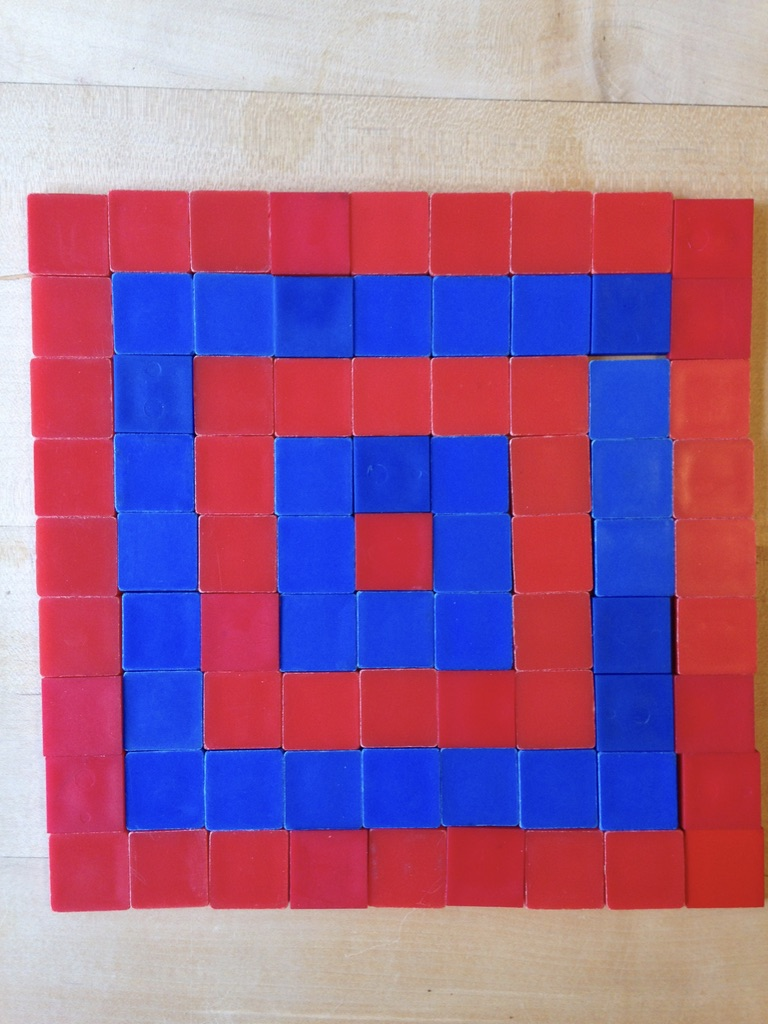Home Free Lessons Square Building##### Description
• Author:
• Posted:
• Topics:
, ,
• Type:
• Keywords:

Topics: Area, multiplication, number patterns , geometry
Materials: Square tiles, graph paper
Common Core: 3.MD.5, 3.MD.6, 3.MD.7, 4.OA.1, 4.NBT.5, 4.MD.3, 5.NBT.5,  MP1, MP2, MP3, MP5, MP6, MP7, MP8

This lesson is truly foundational.
It connects central ideas of arithmetic and geometry, and advances from concrete to pictorial to abstract.
It will prove to be a simple analogy for all variety of future questions.

#### The Launch

This lesson starts easily enough:
you build a few squares out of square tiles, and count how many tiles you need to make each one, and records those numbers.
Then you ask the class: what other numbers of square tiles could you use to build a square?

Write down predictions!
Once students have put forward some guesses,
give them fifteen minutes to build squares of different sizes and write down their list of numbers.

Students may work together as the number of tiles proves insufficient to build the next square.
They can also transition to graph paper and draw out the squares.
The important part is that they build and/or draw the squares, and that they have a written list by the end of the fifteen minutes.

After students have their lists, have them compare them with another classmate to see if they found the same numbers.
Then bring the class together and have the students give you the numbers they found.
They should look like this:

1, 4, 9, 16, 25, 36, 49, 64, 81, 100, …

It’s okay if students don’t get up to the highest numbers.
It’s also okay if students went higher without building every single square.
In fact, it’s great if they start finding patterns that help them predict what the larger square numbers will be.

Important: If there is controversy over which numbers belong on the list, use it.
For example, if some students think that 20 belongs on the list, and others disagree, challenge them prove 20 does or does not belong.

It’s worth mentioning to your students that these numbers, which represent the number of little squares it takes to make a bigger square, are called square numbers. Nifty name, and sensible too.

#### Discussion

Once the numbers are up on the board, ask your students if they see any patterns.
I find that the best way to get responses is to give the class a minute or two to think and to talk to their neighbor about any patterns they see.

And what patterns might they see?
If the kids don’t come up with these, you can challenge them to find them.

Three patterns your students might see:
The numbers go 1 x 1, 2 x 2, 3 x 3, 4 x 4, and so on
The sequence goes Odd, Even, Odd, Even
To get from one number to the next, you go +3, +5, +7, +9

Why do these patterns show up?

I like using a five by five square.
I also like challenging kids to find these patterns.
Give them at least a minute or two to see if they can find each of these patterns.Can you see 5 x 5?
There are five rows of five each, which is a very natural way to see five groups of five.

Can you see 1 + 3 + 5 + 7 + 9?
It’s a nifty way to see how to build up to the next square.

Can you see 1 + 2 + 3 + 4 + 5 + 4 + 3 + 2 +1?
Answer: The trick here is to look on the diagonals.

#### The Wrap

It can be nice to have the students wrap up on a question. Use a student question if a sufficiently compelling issue emerged in the discussion. Depending on how familiar your students are with area and multiplication, you can also consider asking them one or more of these questions to wrap up:

• What is the largest square number you can find?
• True or false: multiplying any square number by 4 gives another square number. Why or why not?
• What is 1 + 3 + 5 + 7 + 9 + 11 + 13 + 15 +17 +19? (How does this relate to squares?)